Using CitectSCADA > Understanding Statistical Process Control > SPC Formulas and Constants

### SPC Formulas and Constants

The SPC calculations are based on the samples collected in subgroups. Each subgroup will have the same number of samples, typically 4. The subgroup size for each SPC tag is set at the SPC Tags properties form.

The number of samples in each subgroup can range from 1 to 25 inclusive.

When the number of samples in each subgroup is 1

Subgroup Mean (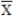):

is the value of the single sample in the group, and is defined by: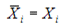Whereis the single sample value in the subgroup.

Moving Range (MR):

is the difference between successive sample values, and is defined by: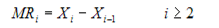Whereis the current sample value and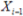is the previous sample value. The number of moving ranges in the process is always one less than the number of subgroups.

Subgroup Standard Deviation (s):

is a measure of absolute variation or dispersion. It describes how much the sample values differ from their mean, and is estimated by: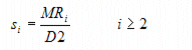The number of subgroup standard deviations in the process is always one less than the number of subgroups.

Process Average (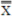):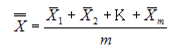Where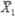,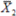, and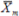are the subgroup means, and m is the total number of subgroups in the process.

Process Range (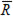):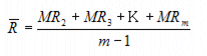Where MR2, MR3, and MRm are the subgroup moving ranges, and m is the total number of subgroups in the process.

Process Standard Deviation (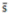):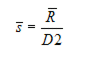When the number of samples in each subgroup is greater than 1

Subgroup Mean ():

is the average (not median or center) of the samples in the group, and is defined by: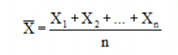Where X1, X2, and Xn are the sample values in the subgroup, and n is the total number of samples in the subgroup.

Subgroup Range (R):

is the difference between the highest and lowest samples in the group, and is defined by: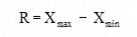Where Xmax is the maximum sample value and Xmin is the minimum sample value in the group.

Subgroup Standard Deviation (s):

is a measure of absolute variation or dispersion. It describes how much the sample values differ from their mean, and is defined by: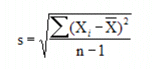Where X's are the sample values in the group,is the group average, and n is the number of samples in the group.

Process Average ():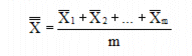Where,, andare the subgroup averages, and m is the total number of subgroups in the process.

Process Range ():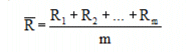Where R1, R2, and Rm are the subgroup ranges, and m is the total number of subgroups in the process.

Process Standard Deviation ():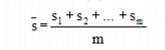Where s1, s2 and sm are the group standard deviations, and m is the total number of groups in the process.

Average Control Limits (UCLx and LCLx):

Specify the approximated 3-sigma boundaries. For a normal distribution 99.74% of the samples will fall within this boundary.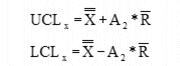Whereis the Process Range and A2 is a constant (given in the Control Chart Line Constants table).

Range Control Limits (UCLR and LCLR):

Specify the approximated 3-sigma boundaries. For a normal distribution 99.74% of the samples will fall within this boundary.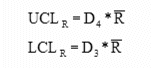Whereis the Process Range and D3 and D4 are constants (given in the Control Chart Line Constants table).

Standard Deviation Control Limits (UCLs and LCLs):

Specify the approximated 3-sigma boundaries. For a normal distribution 99.74% of the samples will fall within this boundary.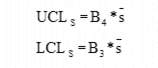Whereis the Process Standard Deviation and B3 and B4 are constants given in the Control Chart Line Constants table).

Process Capability (Cp):

Is the capability of a process to meet a specific tolerance. A process is considered capable when the percentage of samples of a variable for that process that fall within the upper and lower specification limits is greater than a specified value.

The inherent process capability is defined as: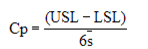• Cp > 1.0 - Indicates the process variation is within the specified limits (USL and LSL) and therefore, is capable.
• Cp < 1.0 - Indicates the process is not capable.

The process capability based on worst case data is defined as: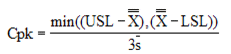• Cpk < 0 - Indicates the process mean is outside the specified limits (USL and LSL)
• Cpk = 0 - Indicates the process mean is equal to one of the specified limits.
• Cpk > 0 - Indicates the process mean is within the specified limits.
• Cpk = 1.0 - Indicates that one side of the 6-sigma limits falls on a specification limit.
• Cpk > 1.0 - Indicates that the 6-sigma limits fall completely within the specified limits.

Skewness (Sk):

Is the degree of asymmetry of a frequency distribution (usually in relation to a normal distribution).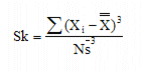where N is the number of samples for the entire process (i.e. Subgroup Size * number of Subgroups).

• Skewness > 0 - Indicates that the histogram's mean (and tail) is pushed to the right.
• Skewness < 0 - Indicates that the histogram's mean (and tail) is pushed to the left.

Kurtosis (Ku):

Is the degree of peakedness of a frequency distribution (usually in relation to a normal distribution).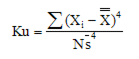where N is the number of samples for the entire process (i.e. Subgroup Size * number of Subgroups).

• Kurtosis < 3 - Indicates a thin distribution with a relatively high peak.
• Kurtosis > 3 - Indicates a distribution that is wide and flat topped.